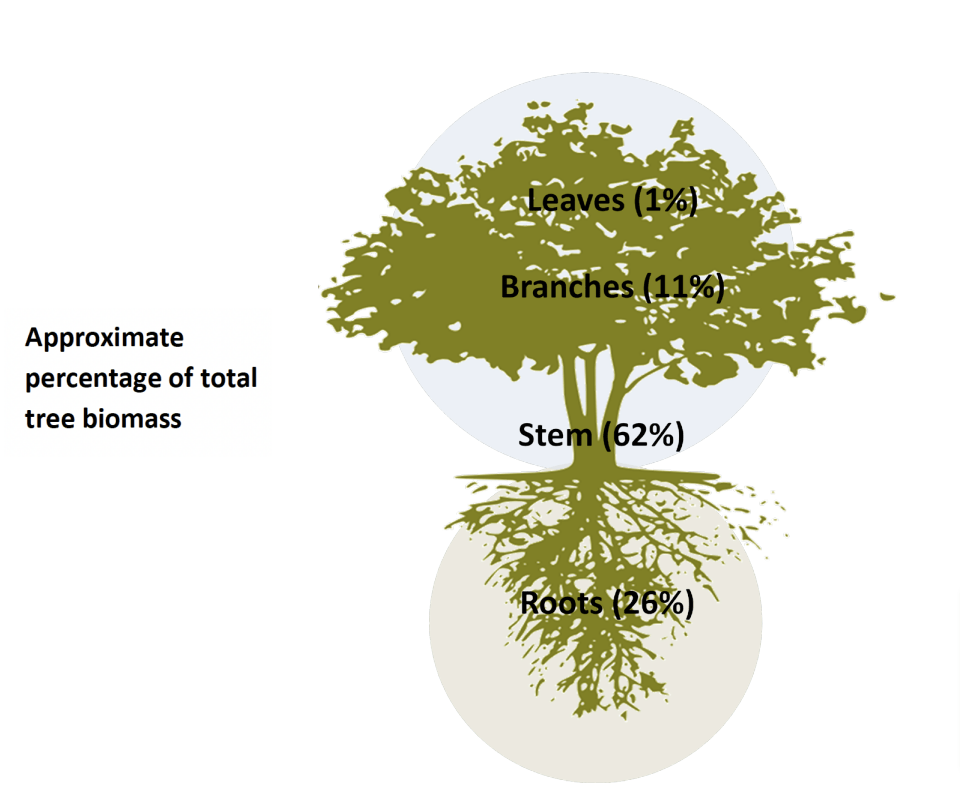At Ecometrica we are often asked, “How many trees do you need to store one tonne of carbon?” The amount of carbon stored by a tree depends on its size, which is influenced by many factors – the species, the local conditions and the way it is managed. In an attempt to find a simple answer to this question we tracked down a tree that stored exactly one tonne of carbon. Here we describe that tree and how we estimated its carbon content.

We found the one tonne carbon tree close to our office in Edinburgh. It is a mature sycamore (Acer pseudoplatanus), with a diameter of 52 cm and a stem height of 12 m. We used standard forestry practices to estimate the amount of carbon contained within the stem, branches, roots and leaves.

To make these estimates we first measured:

• The radius of the stem at 1.3 m above the ground (r1 = 26 cm) and at the top of the stem (r2 = 20 cm), and
• The height of the stem (h = 12 m). These measurements were used to estimate the volume of the stem, from which stem biomass* was calculated. We then used known relationships between the biomass of the stem and the biomass of roots, branches and leaves to estimate the total biomass of the tree.
• Stem volume was estimated using the equation for the volume of a truncated cone:

Volume (m3 ) = 13ℎ(12+2 2+1 ×2)

which gave an estimated stem volume of 2.0 m3.

• The wood density of sycamore is approximately 620 kg/m3 , so using the equation: Mass = Density × Volume the stem biomass was 1243kg, or approximately 1.2 tonnes.
• The biomass of the roots, branches, and leaves of a sycamore tree are known to be around 26%, 11%, and 1% of the total biomass respectively. We used these proportions to estimate the total biomass of the tree without having to dig up roots or cut down branches.So our one tonne carbon tree had a stem with a biomass of 1.2 tonnes, roots of 0.5 tonnes, branches of 0.2 tonnes, and leaves of 0.02 tonnes, giving a total biomass of 2 tonnes. The carbon content of woody matter (stem, branches, and roots) and leaves is approximately 50% of their biomass. Multiplying the total biomass by the proportion of that biomass which is carbon gets us to our estimate of the total amount of carbon stored in the tree. In this case exactly 1 tonne!

### How is carbon sequestered in trees?

As trees photosynthesise they combine carbon dioxide (CO2 ), from the atmosphere with water and nutrients from the ground to form carbohydrates which make up the tree’s biomass. When a tree dies the carbon that is stored in its biomass is either released to the atmosphere or added to the carbon in the soil through decomposition. The rate that carbon is released back to the atmosphere can be controlled by reducing the rate of decomposition, for example by using timber to create long-lived wood products. However, eventually most of the carbon sequestered by the tree will be returned to the atmosphere where each tonne of carbon will be converted to about 3.67 tonnes of carbon dioxide.

A one tonne carbon tree therefore locks up around 3.67 tonnes of carbon dioxide from the atmosphere. The establishment of a natural system of trees in a forest or plantation will ensure that carbon dioxide is kept out of the atmosphere for as long as the forest survives. As individual trees die and return their carbon to the atmosphere they are replaced by new trees through natural regeneration, or further planting.

* “Biomass” is the mass of living matter. This does not include the mass of water present in the plant tissues.

## Need a hard copy?

Author: Ecometrica

Original Post Date: June 2011

This article constitutes a segment of our archival collection, signifying that certain information contained therein may have become obsolete or outdated.